# How to calculate cost basis?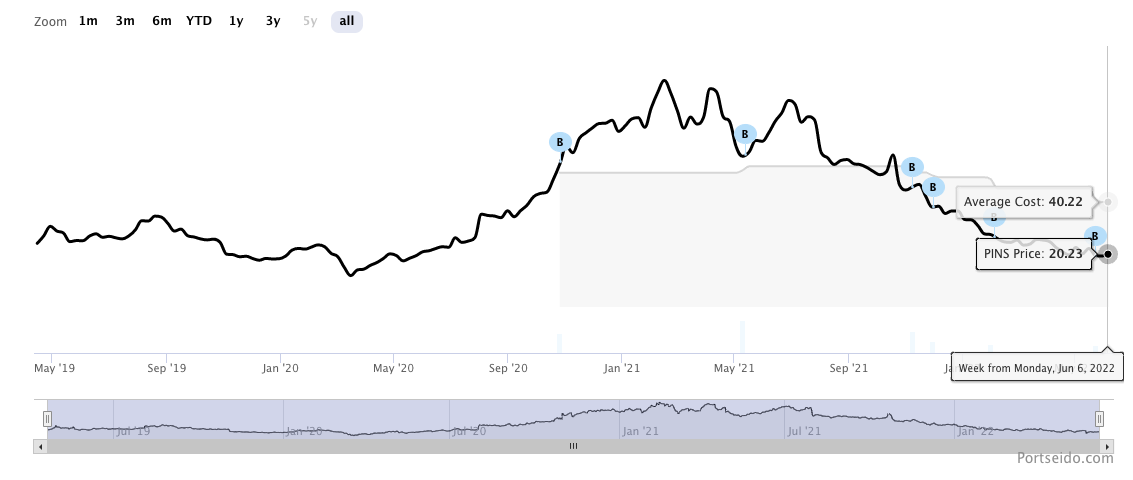If you bought assets (stock, crypto or etfs), average cost would always be one of the main metrics that you looked at. This can be easy and straightforward to calculate. However, things can get complicated when there are multiple buys and sells. In this guide, we attempt to clear out any misconceptions by explaining the two popular methods to calculate cost basis.

## Calculate cost basis

Imagine that you bought 10 shares of AAPL at \$130, then the stock price dropped, and you bought 30 shares more at \$100. Calculating the average price in this case is straightforward. We just need to calculate the weighted average of the price, and use the proportion of the number of shares in each transaction as weight as shown below.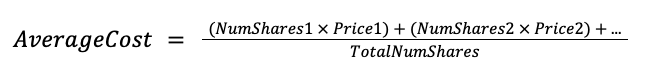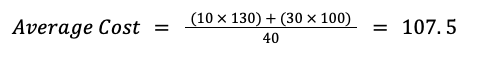## What if I sell?

Imagine the scenario above but now the stock price goes up, and you decide to sell 5 shares of AAPL at \$150. With 35 shares left, what’s your average cost now? To be able to calculate that correctly, we need to choose the method (assumptions) to deal with the shares sold. Each method is still using the average cost formula above. However, they differ on which of the 40 shares have been sold (from the first or the second transaction).

## First In, First Out (FIFO)

As the name says, First In, First Out method (FIFO) assumes that the first shares you have bought got sold first. The calculation is shown below: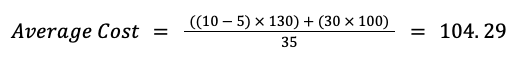As you can see, the 5 shares that have been sold are assumed to be from the first transaction which makes the first transaction (at \$130) left with just 5 shares. While, on the second transaction, it still has the full 30 shares.

## Weighted Average

The Weighted Average method assumes that the shares sold are proportionally from every transaction. Let's illustrate this by using the above example: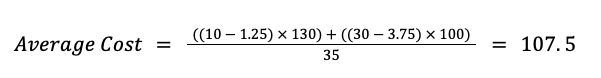By selling 5 shares, this method assumes that 1.25 shares are from the first transaction (25% of 5 shares since the first transaction has 10 shares from the total of 40 shares) and 3.75 shares are from the second one (75% of 5 shares since the second transaction has 30 shares from the total of 40 shares). Therefore, the weights of each transaction before and after selling are the same. This means the sell transaction will have no impact on average cost in weighted average method.

## Other Methods

These two methods are just popular ones among others. In most other cases, the methods just assume which shares to be sold differently. Although Portseido does not support such methods, We think it might be useful to mention it here. Here are a few of them:

• Last In, First Out (LIFO): It’s the opposite of FIFO. The last one coming in is the first one going out.
• High-cost method: The highest price shares got sold first.
• Low-cost method: The lowest price shares gold sold first.

We hope this article helps you figure out the right method for you in calculating average cost. For Portseido user who wants to configure the settings for cost basis, you can do so by following the link here.

PS. Calculating these metrics might cost you a huge amount of time, so don’t waste your time there. Let us calculate them for you at Portseido ;)

Happy investing!